# Rubyplot Tutorial¶

This iruby notebook contains a tutorial and template code for Rubyplot for Magick backend.
P.S. - Tutorial for GR coming soon!

## Step by Step tutorial¶

Any graph in Rubyplot can be created in just 4 easy steps -

### Step 1 - Setting up Rubyplot¶

In :
require 'rubyplot' # Importing Rubyplot
# Set the backend environment variable to desired backend by the command below in the terminal
# export RUBYPLOT_BACKEND="GR"
# export RUBYPLOT_BACKEND="MAGICK"
Rubyplot.set_backend :magick # Choose the backend from magick or gr

# To show the figure in iruby notebook, the function below is called
# Otherwise the figure will be shown in a pop-up window
Rubyplot.inline # showing the figure in iruby notebook
# To stop showing the figure in iruby notebook and instead show in a pop-up window, uncomment and run the next line
# Rubyplot.stop_inline

Out:
true

### Step 2 - Creating the Figure¶

In :
# Declare a new Figure object and specify the height, width and the unit of measurement for the Figure from cm, inch or pixels
figure = Rubyplot::Figure.new(height: 20, width: 20, figsize_unit: :cm) # Declaring a new figure
# Default dimensions are 40 cms

figure.add_subplots! 1,1 # Declaring subplots by specifying the number of rows and columns of subplots in the Figure
# By default a Figure object contains only one subplot, so in case only one subplot is needed, the above code is not needed

# For example, if we want to create a figure with 6 subplots having 3 rows and 2 coulmns, the code is
# The oreintation of the Figure will be -
# |------------|------------|
# |            |            |
# |   (2,0)    |    (2,1)   |
# |            |            |
# |------------|------------|
# |            |            |
# |   (1,0)    |    (1,1)   |
# |            |            |
# |------------|------------|
# |            |            |
# |   (0,0)    |    (0,1)   |
# |            |            |
# |------------|------------|

Out:
[[nil]]

### Step 3 - Declaring the plots¶

To declare plots -

1. Choose the position of the subplot by specifying the position as (row,column) P.S. - The row and column numbers start from 0
2. Choose the type of plot
3. Set the properties of the plot i.e. title, colour, data, etc.
In :
axes00 = figure.add_subplot! 0,0 # Choose the position of the subplot by specifying the row number and column number

# Choose the plot type, here scatter plot is chosen
axes00.scatter! do |p|
# Set the properties of the plot
p.data [1, 2, 3, 4, 5],[12, 55, 4, 10, 24] # Data as arrays of x coordinates and y coordinates
# i.e. the points are (1,12), (2,55), (3,4), (4,10), (5,24)
p.marker_type = :diamond # Type of marker
p.marker_fill_color = :lemon # Colour to be filled inside the marker
p.marker_size = 2 # Size of the marker, unit is 15*pixels
p.marker_border_color = :black # Colour of the border of the marker
p.label = "Diamonds" # Label for this data
end

# Set the properties of the subplot
axes00.title = "A scatter plot" # Title of the plot
axes00.x_title = "X-axis" # Title of the X axis
axes00.y_title = "Y-axis" # Title of the Y-axis
axes00.square_axes = true # Setting square axes

# If there are multiple subplots, repeat this step for each subplot

Out:
true

### Step 4 - Save or display the Figure¶

#### Display¶

In :
# Displaying the Figure by calling the show function
figure.show # Since inline was already called, the figure is displayed in the iruby notebook

Out: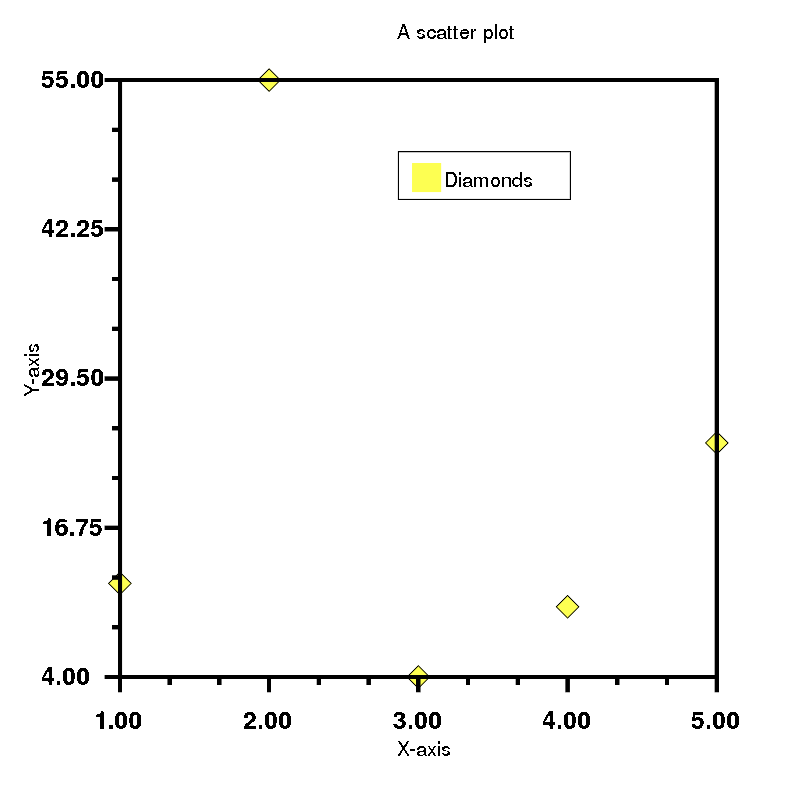In [ ]:
# Displaying in a pop-up window
Rubyplot.stop_inline # Stopping inline display
figure.show # Calling show function for displaying the Figure

Rubyplot.inline # Reverting back to inline for convenience in the tutorial


#### Saving in a file¶

In [ ]:
# Calling the write function to save the Figure
figure.write('./1.png') # Input is a relative or absolute path with image format specified


## Examples¶

Plot function-
18. Plot function Examples

### 1. Scatter plot¶

In :
# Step 1
require 'rubyplot'
Rubyplot.set_backend :magick
Rubyplot.inline

# Step 2
figure = Rubyplot::Figure.new(width: 20, height: 20)

# Step 3
axes00.scatter! do |p|
p.data [1, 2, 3, 4, 5],[12, 55, 4, 10, 24] # Data as arrays of x coordinates and y coordinates
# i.e. the points are (1,12), (2,55), (3,4), (4,10), (5,24)
p.marker_type = :diamond # Type of marker
p.marker_fill_color = :lemon # Colour to be filled inside the marker
p.marker_size = 2 # Size of the marker, unit is 15*pixels
p.marker_border_color = :black # Colour of the border of the marker
p.label = "Diamonds"# Label for this data
end

axes00.title = "A scatter plot"
axes00.x_title = "X-axis"
axes00.y_title = "Y-axis"
axes00.square_axes = false

# Step 4
figure.show

Out: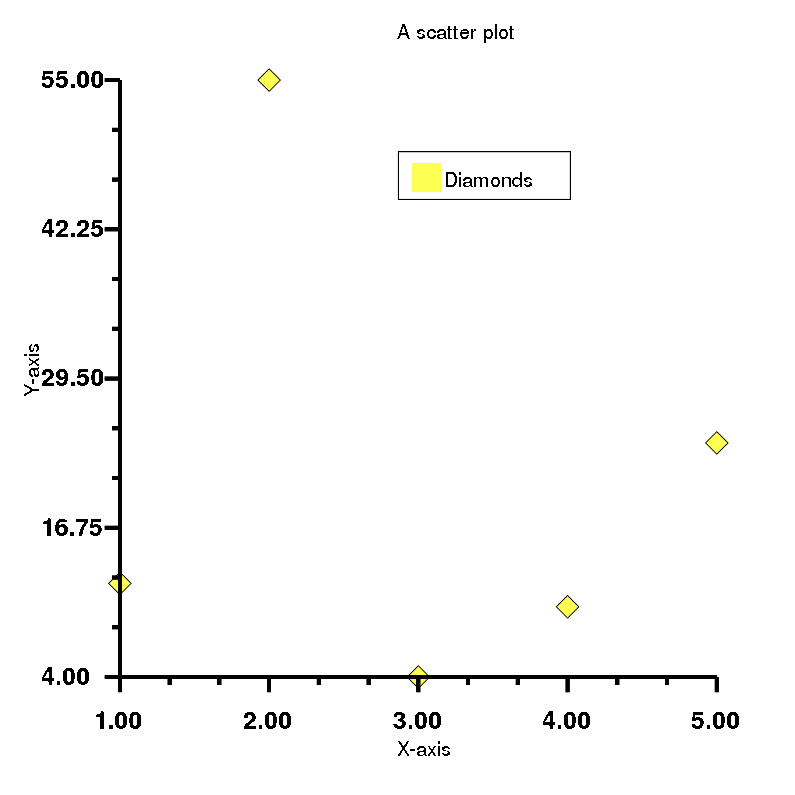### 2. Bar plot¶

In :
# Step 1
require 'rubyplot'
Rubyplot.set_backend :magick
Rubyplot.inline

# Step 2
figure = Rubyplot::Figure.new(width: 20, height: 20)

# Step 3
axes00.bar! do |p|
p.data [23, 13, 45, 67, 5] # Data as given as heights of bars
p.color = :neon_red # Colour of the bars
p.spacing_ratio = 0.3 # Ratio of space the bars don't occupy out of the maximum space allotted to each bar
# Each bar is allotted equal space, so maximum space for each bar is total space divided by the number of bars
p.label = "Points"# Label for this data
end

axes00.title = "A bar plot"
axes00.x_title = "X-axis"
axes00.y_title = "Y-axis"
axes00.square_axes = false

# Step 4
figure.show

Out: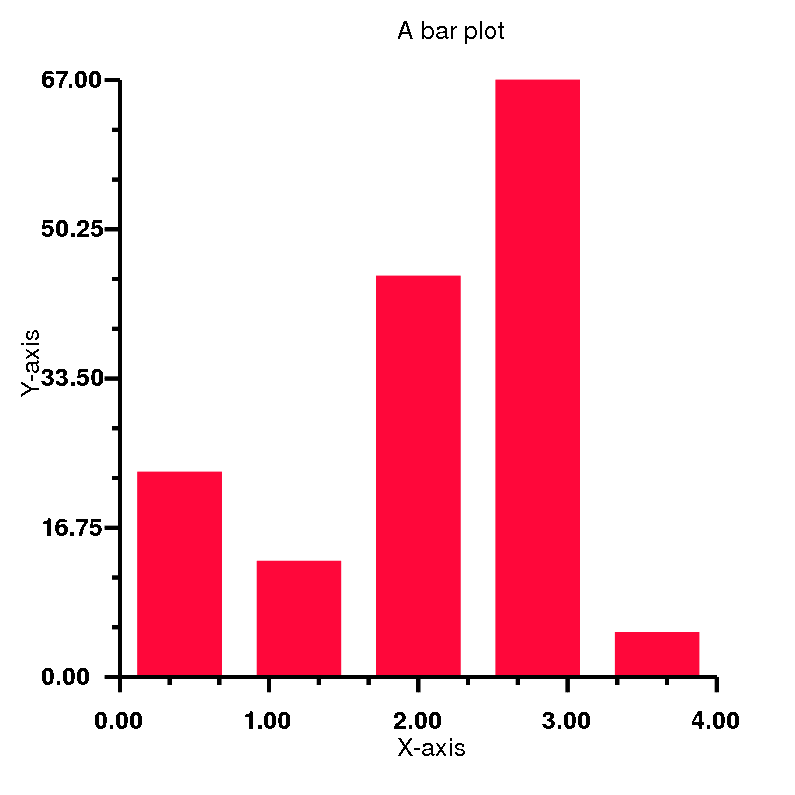### 3. Area plot¶

In :
# Step 1
require 'rubyplot'
Rubyplot.set_backend :magick
Rubyplot.inline

# Step 2
figure = Rubyplot::Figure.new(width: 20, height: 20)

# Step 3
axes00.area! do |p|
p.data [1, 2, 3, 4, 5, 6], [3, 2, 5, 5, 7,4] # Data as x coordinate values, height of consecutive points i.e. y coordinates
p.color = :orange # Color of the area
p.label = "Stock A"# Label for this data
p.stacked false # stacked option makes area plot opaque i.e. opacity = 1
# Opacity of the area plot is set to 0.3 for visibility if not stacked
end

axes00.title = "An area plot"
axes00.x_title = "Time"
axes00.y_title = "Value"
axes00.square_axes = false

# Step 4
figure.show

Out: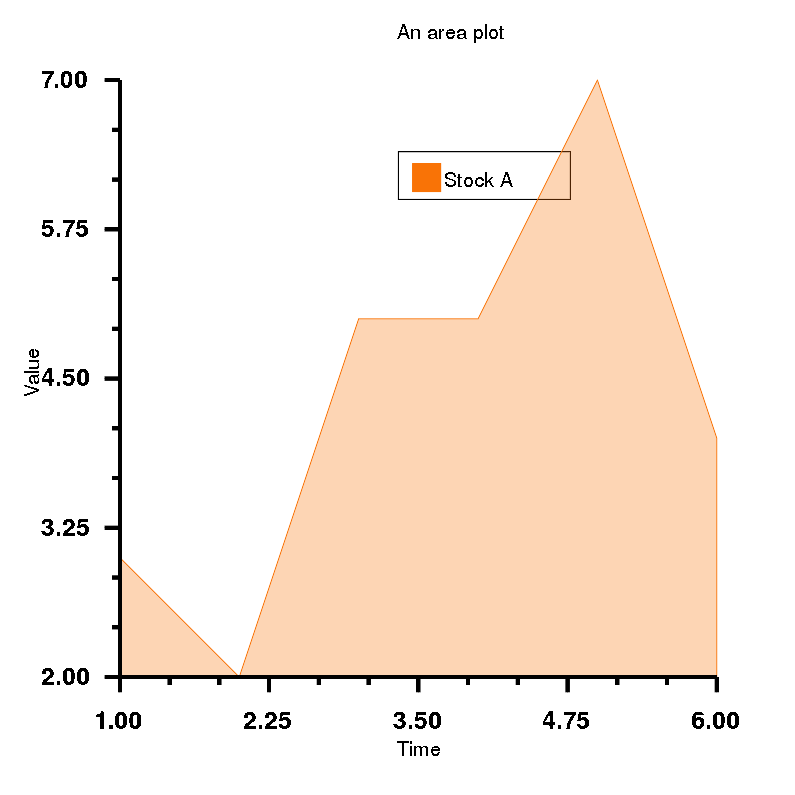In :
# Data input format may be changed in the near future (18th Aug 2019)

# Step 1
require 'rubyplot'
Rubyplot.set_backend :magick
Rubyplot.inline

# Step 2
figure = Rubyplot::Figure.new(width: 20, height: 20)

# Step 3
axes00.area! do |p|
p.data [1, 2, 3, 4, 5, 6], [3, 2, 5, 5, 7, 4] # Data as height of consecutive points i.e. y coordinates
p.color = :black # Color of the area
p.label = "Stock A"# Label for this data
p.stacked true # stacked option makes area plot opaque i.e. opacity = 1
# Opacity of the area plot is set to 0.3 for visibility if not stacked
end
axes00.area! do |p|
p.data [1, 2, 3, 4, 5, 6], [2, 1, 3, 4, 5, 1] # Data as height of consecutive points i.e. y coordinates
p.color = :yellow # Color of the area
p.label = "Stock B"# Label for this data
p.stacked true # stacked option makes area plot opaque i.e. opacity = 1
# Opacity of the area plot is set to 0.3 for visibility if not stacked
end

axes00.title = "An area plot"
axes00.x_title = "Time"
axes00.y_title = "Value"
axes00.square_axes = false

# Step 4
figure.show

Out: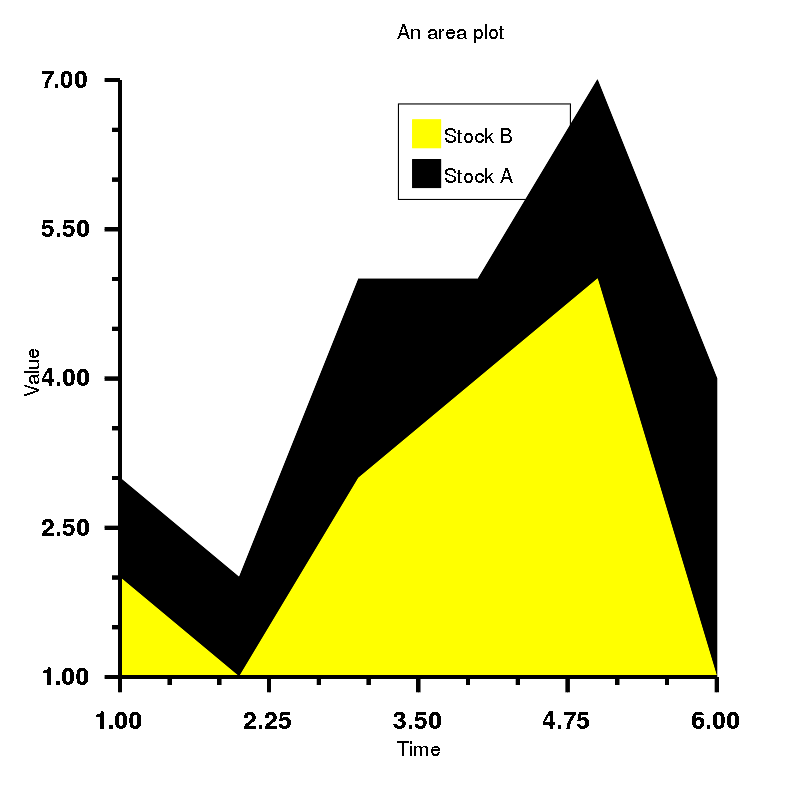### 4. Bubble plot¶

In :
# Step 1
require 'rubyplot'
Rubyplot.set_backend :magick
Rubyplot.inline

# Step 2
figure = Rubyplot::Figure.new(width: 20, height: 20)

# Step 3
axes00.bubble! do |p|
p.data [12, 4, 25, 7, 19], [50, 30, 75, 12, 25], [0.5, 0.7, 0.4, 0.5, 1] # Data as arrays of x coordinates, y coordinates and sizes
# Size units are 27.5*pixel
p.color = :blue # Colour of the bubbles
p.label = "Bubbles"# Label for this data
p.fill_opacity = 0.7 # Opacity of the bubbles, default = 0.5
end

axes00.title = "A bubble plot"
axes00.x_title = "X-axis"
axes00.y_title = "Y-axis"
axes00.square_axes = false

# Step 4
figure.show

Out: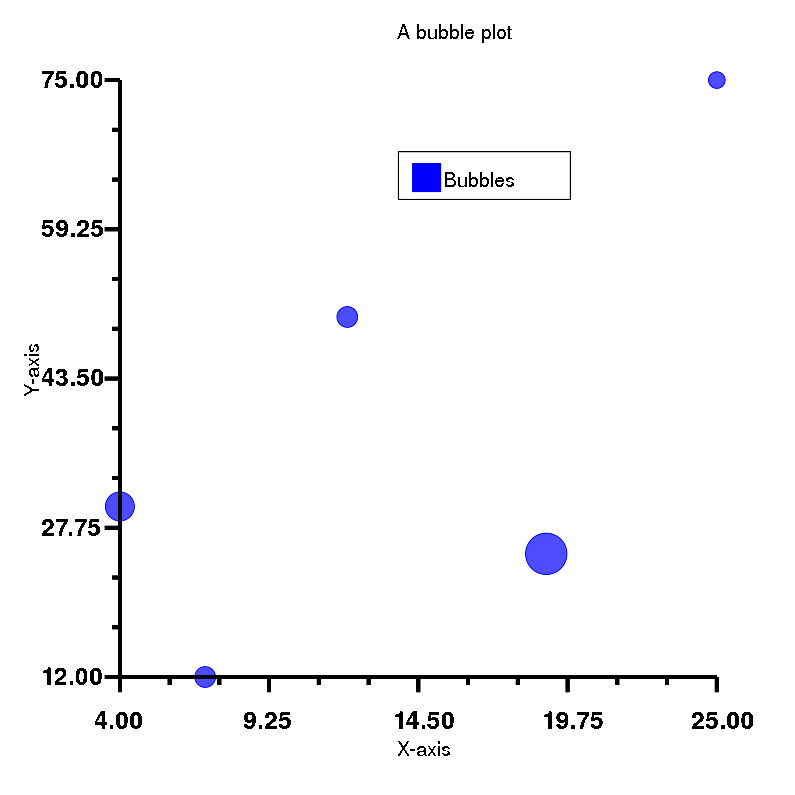In :
# Step 1
require 'rubyplot'
Rubyplot.set_backend :magick
Rubyplot.inline

# Step 2
figure = Rubyplot::Figure.new(width: 20, height: 20)

# Step 3
axes00.bubble! do |p|
p.data [12, 4, 25, 7, 19], [50, 30, 75, 12, 25], [0.5, 0.7, 0.4, 0.5, 1] # Data as arrays of x coordinates, y coordinates and sizes
# Size units are 27.5*pixel
p.color = :blue # Colour of the bubbles
p.label = "Bubbles 1"# Label for this data
# Opacity of the bubbles is set to 0.5 for visibility
end
axes00.bubble! do |p|
p.data [1, 7, 20, 27, 17], [41, 30, 48, 22, 5], [0.5, 1, 0.8, 0.9, 1] # Data as arrays of x coordinates, y coordinates and sizes
# Size units are 27.5*pixel
p.color = :red # Colour of the bubbles
p.label = "Bubbles 2"# Label for this data
# Opacity of the bubbles is set to 0.5 for visibility
end

axes00.title = "A bubble plot"
axes00.x_title = "X-axis"
axes00.y_title = "Y-axis"
axes00.square_axes = false

# Step 4
figure.show

Out: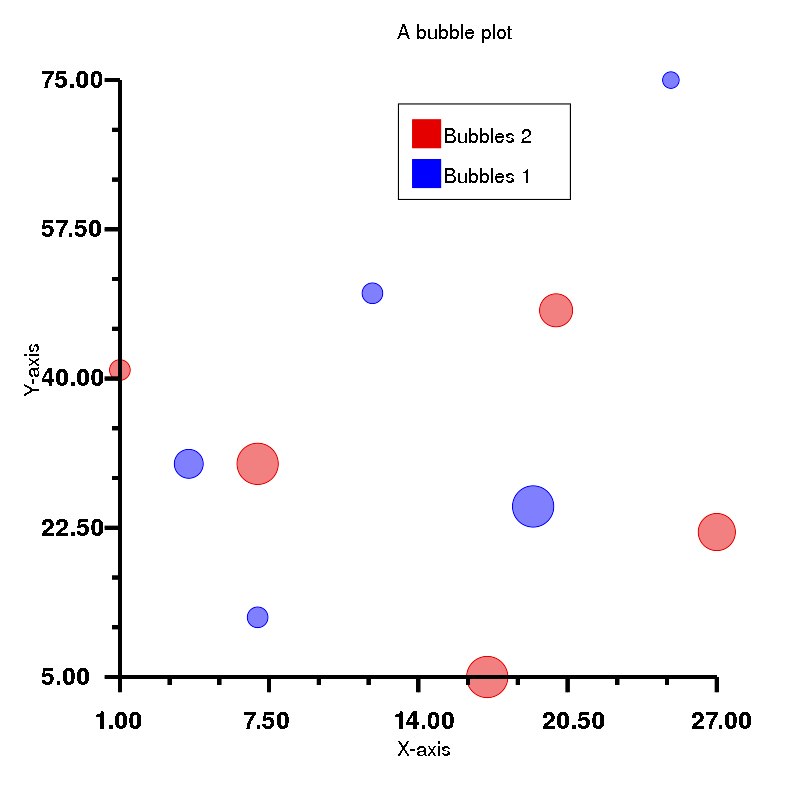### 5. Histogram¶

In :
# Step 1
require 'rubyplot'
Rubyplot.set_backend :magick
Rubyplot.inline

# Step 2
figure = Rubyplot::Figure.new(width: 20, height: 20)

# Step 3
axes00.histogram! do |p|
p.data 100.times.map{ rand(10) } # Data as an array of values
p.color = :electric_lime # Colour of the bars
p.label = "Counts"# Label for this data
# bins are not given so they are decided by Rubyplot
end

axes00.title = "A histogram"
axes00.x_title = "X-axis"
axes00.y_title = "Y-axis"
axes00.square_axes = false

# Step 4
figure.show

Out: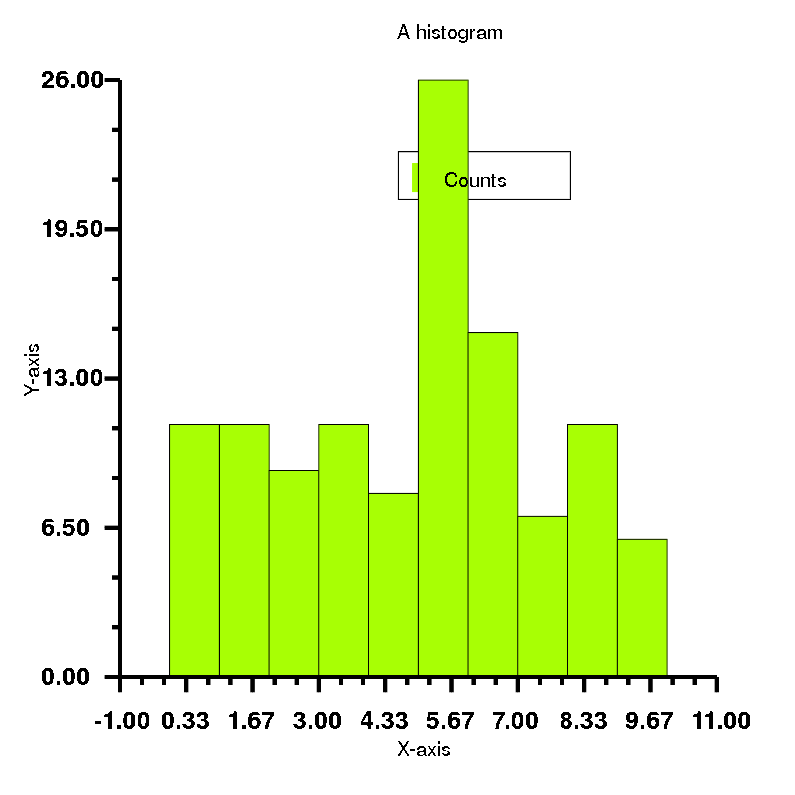In [ ]:
# To be corrected

# Step 1
require 'rubyplot'
Rubyplot.set_backend :magick
Rubyplot.inline

# Step 2
figure = Rubyplot::Figure.new(width: 20, height: 20)

# Step 3
axes00.histogram! do |p|
p.x = 150.times.map{ rand(10) } # Data as an array of values
p.color = :electric_lime # Colour of the bars
p.label = "Counts"# Label for this data
p.bins = 2 # An integer specifying the number of bins is given
end

axes00.title = "A histogram"
axes00.x_title = "X-axis"
axes00.y_title = "Y-axis"
axes00.square_axes = true

# Step 4
# figure.show

In :
# Step 1
require 'rubyplot'
Rubyplot.set_backend :magick
Rubyplot.inline

# Step 2
figure = Rubyplot::Figure.new(width: 20, height: 20)

# Step 3
axes00.histogram! do |p|
p.x = 100.times.map{ rand(10) } # Data as an array of values
p.color = :electric_lime # Colour of the bars
p.label = "Counts"# Label for this data
p.bins = [1, 4, 7, 10] # bins given directly i.e. bins are [1,4), [4,7), [7,10]
end

axes00.title = "A histogram"
axes00.x_title = "X-axis"
axes00.y_title = "Y-axis"
axes00.square_axes = true

# Step 4
figure.show

Out: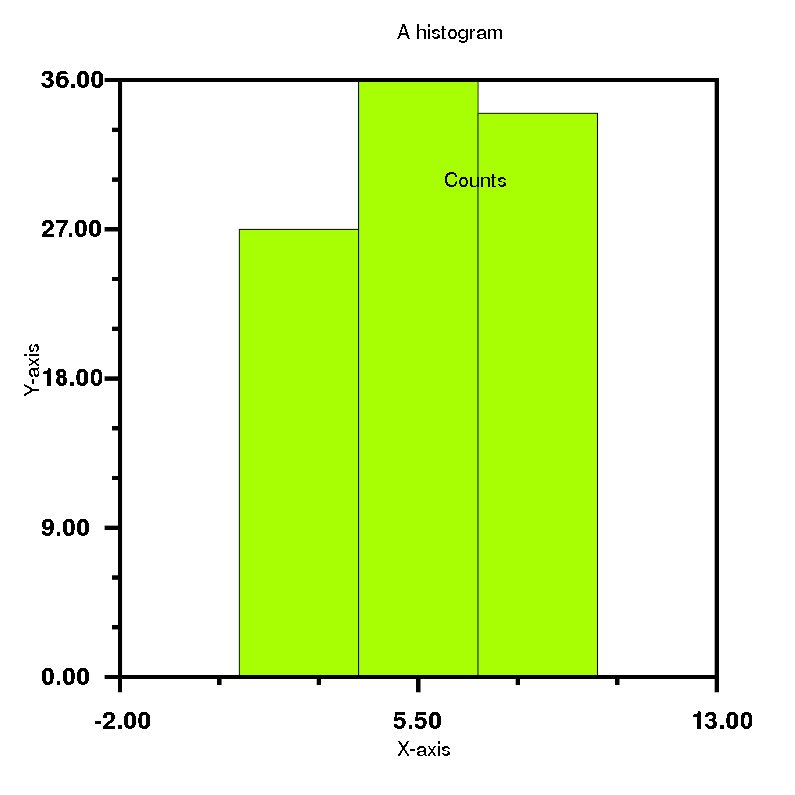### 6. Candle-stick plot¶

In :
# Step 1
require 'rubyplot'
Rubyplot.set_backend :magick
Rubyplot.inline

# Step 2
figure = Rubyplot::Figure.new(width: 20, height: 20)

# Step 3
axes00.candle_stick! do |p|
p.lows = [100, 110, 120, 130, 120, 110] # Array for minimum values for sticks
p.highs = [140, 150, 160, 170, 160, 150] # Array for maximum value for sticks
p.opens = [110, 120, 130, 140, 130, 120] # Array for minimum value for bars
p.closes = [130, 140, 150, 160, 150, 140] # Array for maximum value for bars
p.border_color = :black # Colour of the border of the bars
p.color = :yellow # Colour of the bars
p.label = "Data"# Label for this data
end

axes00.title = "A candle-stick plot"
axes00.x_title = "X-axis"
axes00.y_title = "Y-axis"
axes00.square_axes = false

# Step 4
figure.show

Out: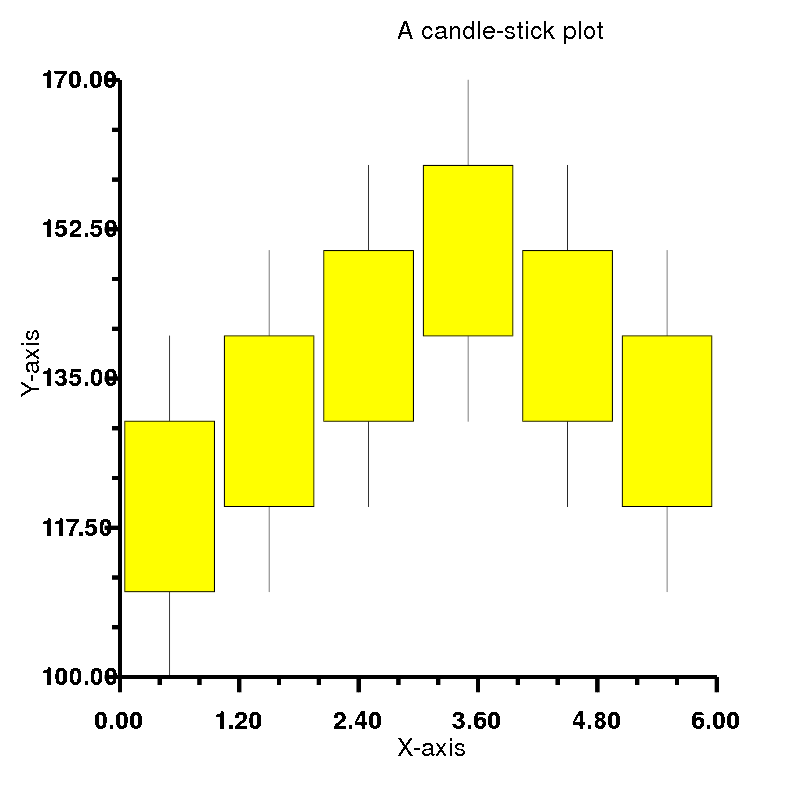### 7. Error-bar plot¶

In :
# Step 1
require 'rubyplot'
Rubyplot.set_backend :magick
Rubyplot.inline

# Step 2
figure = Rubyplot::Figure.new(width: 20, height: 20)

# Step 3
axes00.error_bar! do |p|
p.data [1,2,3,4], [1,4,9,16] # Arrays for x coordinates and y coordinates
p.xerr = [0.5,1.0,1.5,0.3] # X error for each point
p.yerr = [0.6,0.2,0.8,0.1] # Y error for each point
p.color = :red # Colour of the line
p.label = "Values"# Label for this data
end

axes00.title = "An error-bar plot"
axes00.x_title = "X-axis"
axes00.y_title = "Y-axis"
axes00.square_axes = false

# Step 4
figure.show

Out: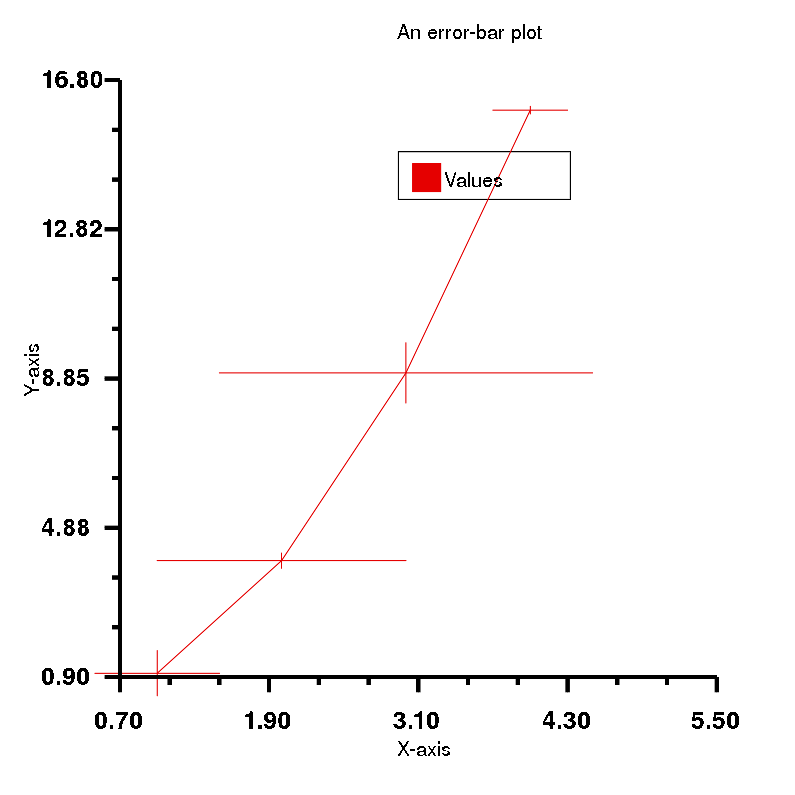### 8. Box plot¶

In :
# Step 1
require 'rubyplot'
Rubyplot.set_backend :magick
Rubyplot.inline

# Step 2
figure = Rubyplot::Figure.new(width: 20, height: 20)

# Step 3
axes00.box_plot! do |p|
p.data [
[60,70,80,70,50],
[100,40,20,80,70],
[30, 10]
] # Array of arrays for data for each box
p.color = :blue # Colours of the boxes
p.whiskers = 0.3 # whiskers for determining outliers
p.outlier_marker_type = :hglass # Type of the outlier marker
p.outlier_marker_color = :yellow # Fill colour of the outlier marker
# Border colour of the outlier marker is set to black
p.outlier_marker_size = 1 # Size of the outlier marker
p.label = "Data"# Label for this data
end

axes00.title = "A box plot"
axes00.x_title = "X-axis"
axes00.y_title = "Y-axis"
axes00.square_axes = false

# Step 4
figure.show

Out: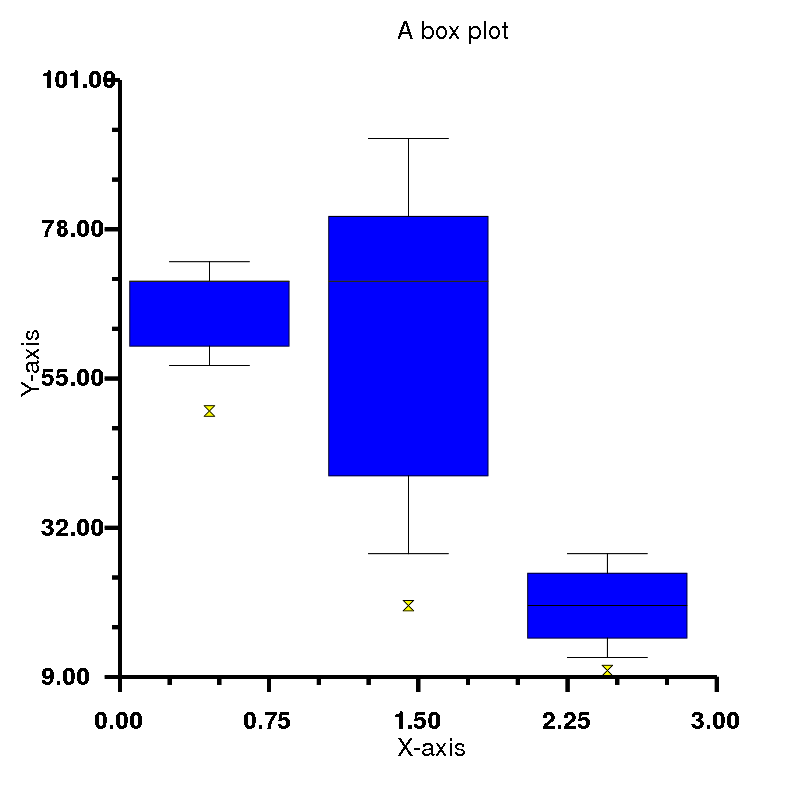### 9. Line plot¶

In :
# Step 1
require 'rubyplot'
Rubyplot.set_backend :magick
Rubyplot.inline

# Step 2
figure = Rubyplot::Figure.new(width: 20, height: 20)

# Step 3
axes00.line! do |p|
p.data [1, 2, 3, 4, 5],[12, 55, 4, 10, 24] # Data as arrays for values of x coordinates, y coordinates
p.line_type = :solid # Type of the line
p.line_color = :yellow # Colour of the line
p.line_width = 2 # Width of the line
p.label = "Values"# Label for this data
end

axes00.title = "A line plot"
axes00.x_title = "X-axis"
axes00.y_title = "Y-axis"
axes00.square_axes = false

# Step 4
figure.show

Out: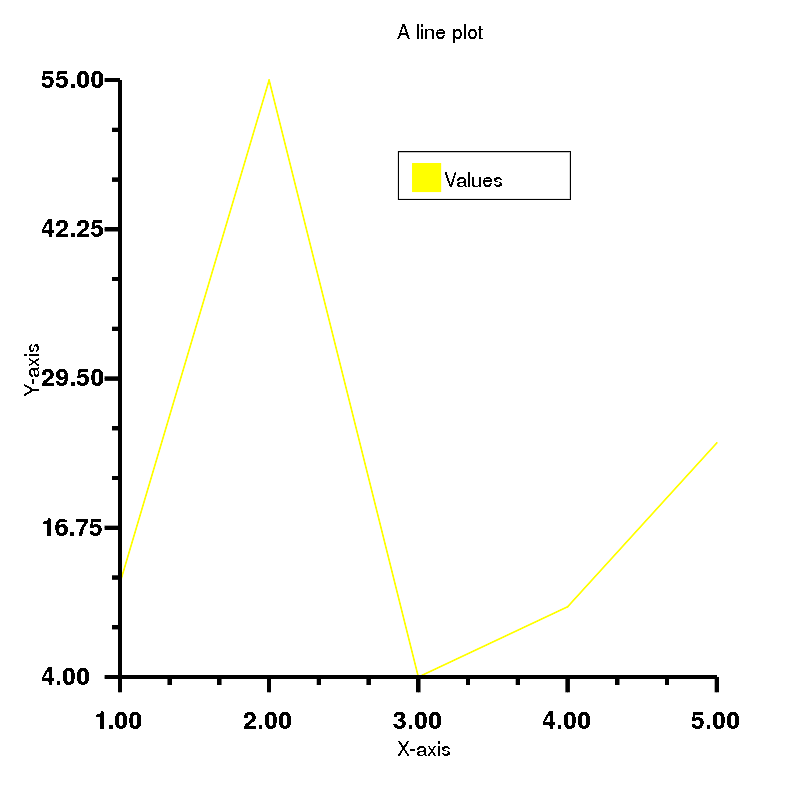In :
# Colour of multiple lines will be corrected

# Step 1
require 'rubyplot'
Rubyplot.set_backend :magick
Rubyplot.inline

# Step 2
figure = Rubyplot::Figure.new(width: 20, height: 20)

# Step 3
axes00.line! do |p|
p.data [1, 2, 3, 4, 5], [10, 20, 30, 40, 50] # Data as arrays for values of x coordinates, y coordinates
p.line_type = :solid # Type of the line
p.line_color = :blue # Colour of the line
p.line_width = 2 # Width of the line
p.label = "Line 1"# Label for this data
end
axes00.line! do |p|
p.data [2, 3, 4, 5, 6], [5, 55, 23, 10, 49] # Data as arrays for values of y coordinates, x coordinates
p.line_type = :solid # Type of the line
p.line_color = :yellow # Colour of the line
p.line_width = 2 # Width of the line
p.label = "Line 2"# Label for this data
end

axes00.title = "A line plot"
axes00.x_title = "X-axis"
axes00.y_title = "Y-axis"
axes00.square_axes = false

# Step 4
figure.show

Out: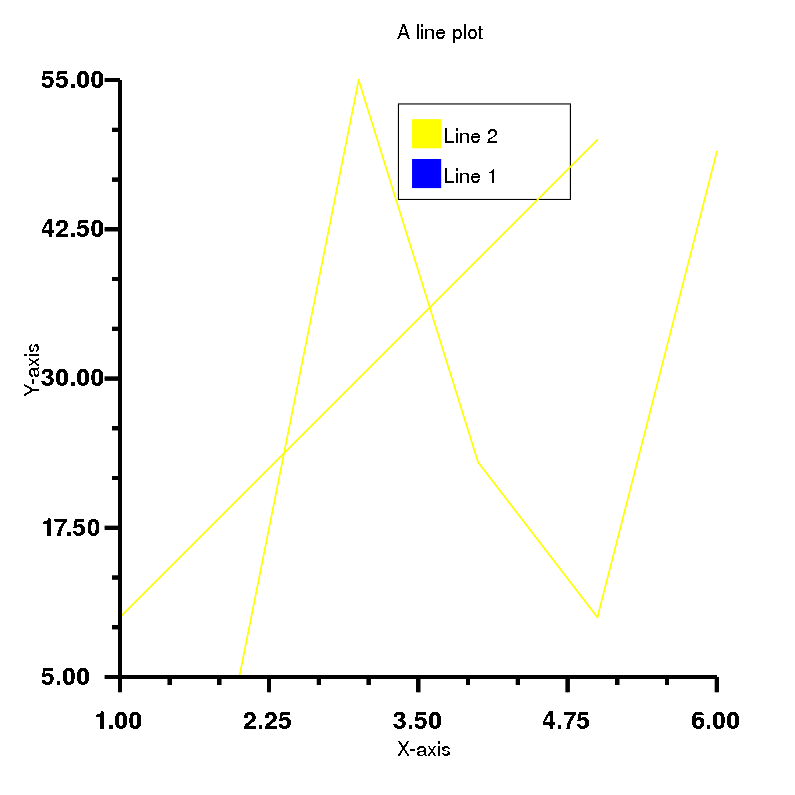### 10. Stacked-bar plot¶

In :
# A stacked bar plot with just one set of values looks same as a bar plot

# Step 1
require 'rubyplot'
Rubyplot.set_backend :magick
Rubyplot.inline

# Step 2
figure = Rubyplot::Figure.new(width: 20, height: 20)

# Step 3
axes00.stacked_bar! do |p|
p.data [1, 2, 3, 4, 5] # Data as height of bars
p.color = :lemon # Colour of the bars
p.spacing_ratio = 0.2 # Ratio of space the bars don't occupy out of the maximum space allotted to each bar
# Each bar is allotted equal space, so maximum space for each bar is total space divided by the number of bars
p.label = "Diamonds"# Label for this data
end

axes00.title = "A stacked-bar plot"
axes00.x_title = "X-axis"
axes00.y_title = "Y-axis"
axes00.square_axes = false

# Step 4
figure.show

Out: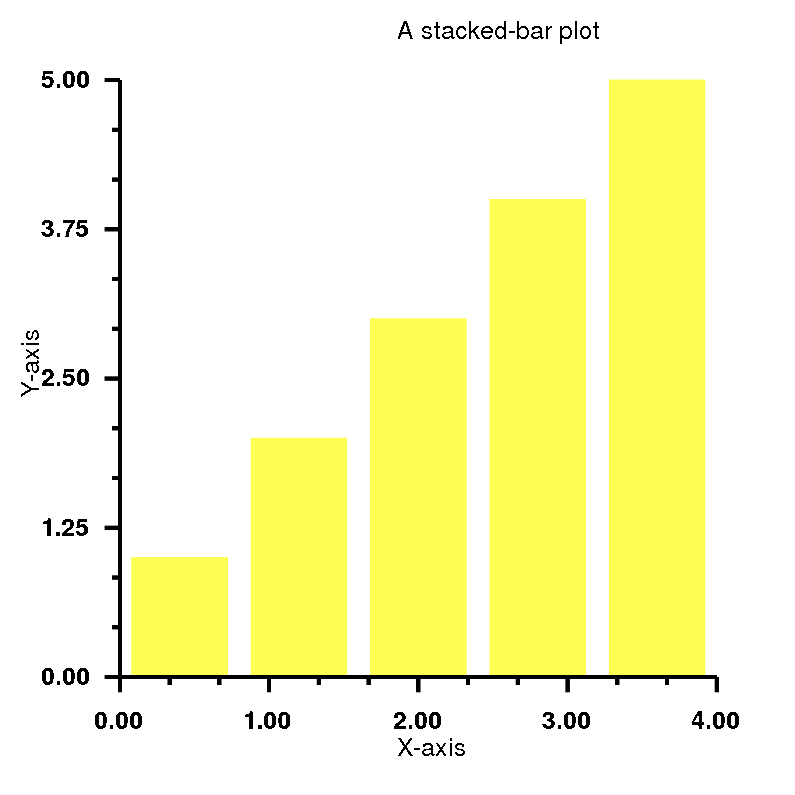### 11. Multi Stacked-bar plot¶

In :
# Step 1
require 'rubyplot'
Rubyplot.set_backend :magick
Rubyplot.inline

# Step 2
figure = Rubyplot::Figure.new(width: 20, height: 20)

# Step 3
axes00.stacked_bar! do |p|
p.data [1, 2, 3, 4, 5] # Data as height of bars
p.color = :lemon # Colour of the bars
p.spacing_ratio = 0.2 # Ratio of space the bars don't occupy out of the maximum space allotted to each bar
# Each bar is allotted equal space, so maximum space for each bar is total space divided by the number of bars
p.label = "Stock 1"# Label for this data
end
# Spacing ratio declared first is considered
axes00.stacked_bar! do |p|
p.data [5, 4, 3, 2, 1] # Data as height of bars
p.color = :blue # Colour of the bars
p.spacing_ratio = 0.2 # Ratio of space the bars don't occupy out of the maximum space allotted to each bar
# Each bar is allotted equal space, so maximum space for each bar is total space divided by the number of bars
p.label = "Stock 2"# Label for this data
end
axes00.stacked_bar! do |p|
p.data [3, 5, 7, 5, 3] # Data as height of bars
p.color = :red # Colour of the bars
p.spacing_ratio = 0.2 # Ratio of space the bars don't occupy out of the maximum space allotted to each bar
# Each bar is allotted equal space, so maximum space for each bar is total space divided by the number of bars
p.label = "Stock 3"# Label for this data
end

axes00.title = "A multi stacked-bar plot"
axes00.x_title = "X-axis"
axes00.y_title = "Y-axis"
axes00.square_axes = false

# Step 4
figure.show

Out: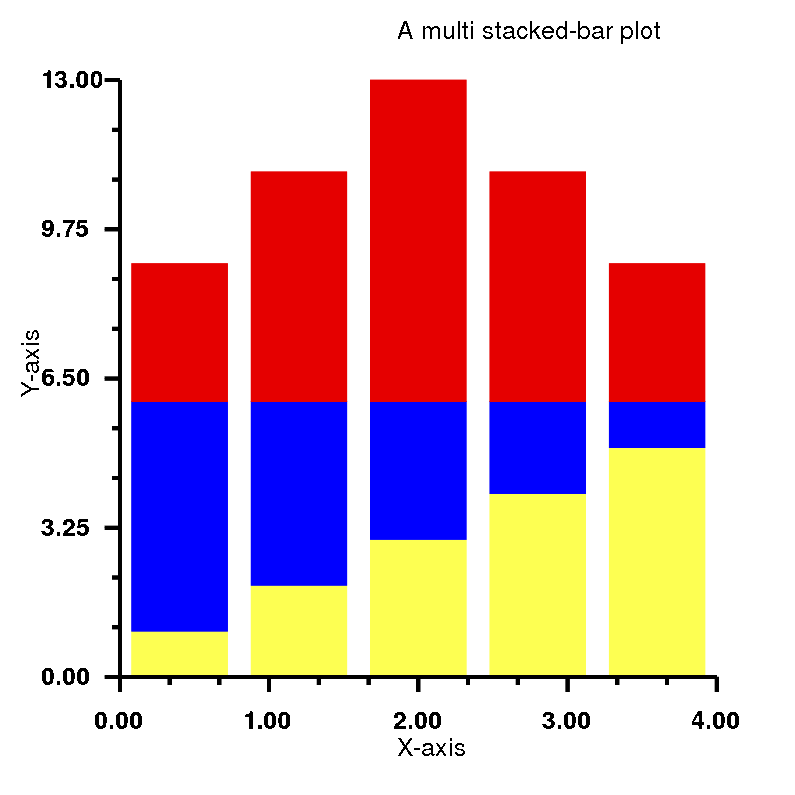### 12. Multi Bar plot¶

In :
# Step 1
require 'rubyplot'
Rubyplot.set_backend :magick
Rubyplot.inline

# Step 2
figure = Rubyplot::Figure.new(width: 20, height: 20)

# Step 3
axes00.bar! do |p|
p.data [1, 2, 3, 4, 5] # Data as height of bars
p.color = :lemon # Colour of the bars
p.spacing_ratio = 0.2 # Ratio of space the bars don't occupy out of the maximum space allotted to each bar
# Each bar is allotted equal space, so maximum space for each bar is total space divided by the number of bars
p.label = "Stock 1"# Label for this data
end
# Spacing ratio declared first is considered
axes00.bar! do |p|
p.data [5, 4, 3, 2, 1] # Data as height of bars
p.color = :blue # Colour of the bars
p.spacing_ratio = 0.2 # Ratio of space the bars don't occupy out of the maximum space allotted to each bar
# Each bar is allotted equal space, so maximum space for each bar is total space divided by the number of bars
p.label = "Stock 2"# Label for this data
end
axes00.bar! do |p|
p.data [3, 5, 7, 5, 3] # Data as height of bars
p.color = :red # Colour of the bars
p.spacing_ratio = 0.2 # Ratio of space the bars don't occupy out of the maximum space allotted to each bar
# Each bar is allotted equal space, so maximum space for each bar is total space divided by the number of bars
p.label = "Stock 3"# Label for this data
end

axes00.title = "A multi bar plot"
axes00.x_title = "X-axis"
axes00.y_title = "Y-axis"
axes00.square_axes = false

# Step 4
figure.show

Out: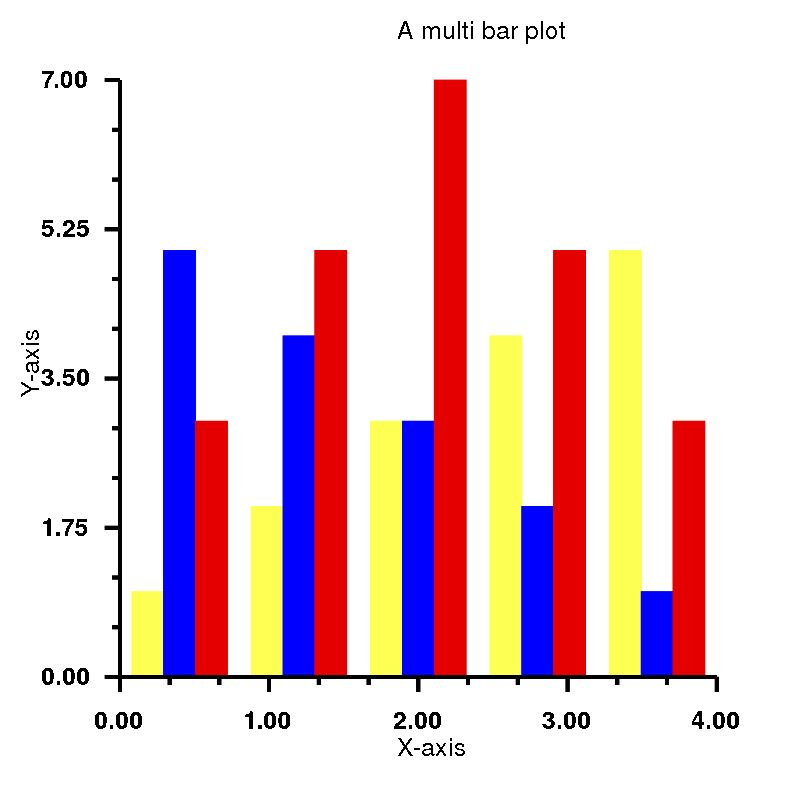### 13. Multi Candle-stick plot¶

In :
# Step 1
require 'rubyplot'
Rubyplot.set_backend :magick
Rubyplot.inline

# Step 2
figure = Rubyplot::Figure.new(width: 20, height: 20)

# Step 3
axes00.candle_stick! do |p|
p.lows = [100, 110, 120, 130, 120, 110] # Array for minimum values for sticks
p.highs = [140, 150, 160, 170, 160, 150] # Array for maximum value for sticks
p.opens = [110, 120, 130, 140, 130, 120] # Array for minimum value for bars
p.closes = [130, 140, 150, 160, 150, 140] # Array for maximum value for bars
p.border_color = :black # Colour of the border of the bars
p.color = :yellow # Colour of the bars
p.label = "Data 1"# Label for this data
end
axes00.candle_stick! do |p|
p.lows = [105, 105, 125, 130, 110, 110] # Array for minimum values for sticks
p.highs = [150, 140, 165, 170, 180, 160] # Array for maximum value for sticks
p.opens = [110, 120, 130, 140, 130, 120] # Array for minimum value for bars
p.closes = [135, 135, 145, 160, 175, 150] # Array for maximum value for bars
p.border_color = :red # Colour of the border of the bars
p.color = :blue # Colour of the bars
p.label = "Data 2"# Label for this data
end

axes00.title = "A multi candle-stick plot"
axes00.x_title = "X-axis"
axes00.y_title = "Y-axis"
axes00.square_axes = false

# Step 4
figure.show

Out: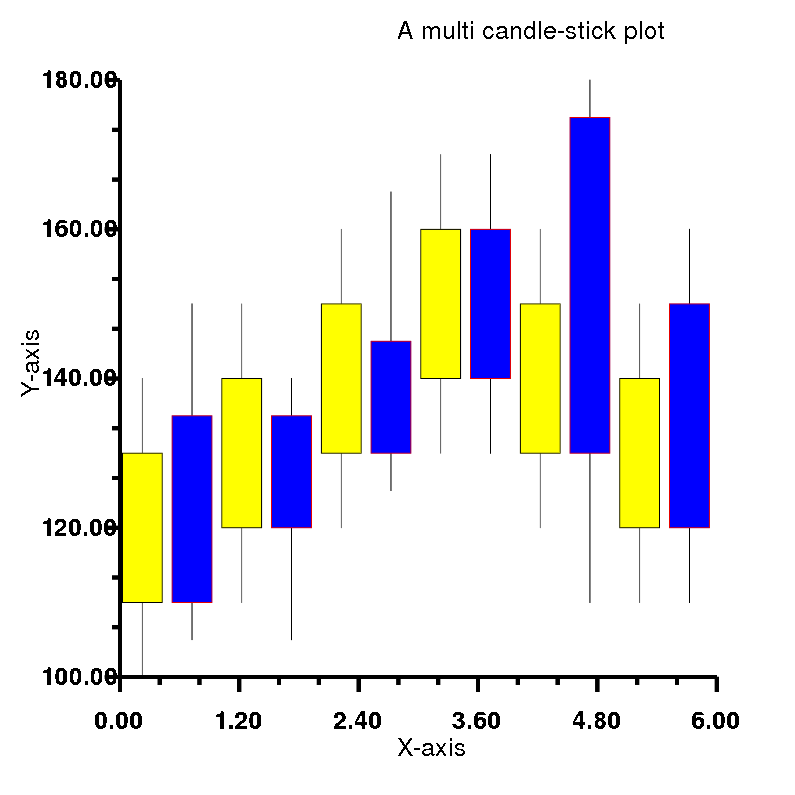### 14. Multi Box plot¶

In :
# Step 1
require 'rubyplot'
Rubyplot.set_backend :magick
Rubyplot.inline

# Step 2
figure = Rubyplot::Figure.new(width: 20, height: 20)

# Step 3
axes00.box_plot! do |p|
p.data [
[60,70,80,70,50],
[100,40,20,80,70],
[30, 10]
] # Array of arrays for data for each box
p.color = :lemon # Colours of the boxes
p.whiskers = 0.3 # whiskers for determining outliers
p.outlier_marker_type = :hglass # Type of the outlier marker
p.outlier_marker_color = :yellow # Fill colour of the outlier marker
# Border colour of the outlier marker is set to black
p.outlier_marker_size = 1 # Size of the outlier marker
p.label = "Data"# Label for this data
end
axes00.box_plot! do |p|
p.data [
[10, 30, 90, 30, 20],
[120, 140, 150, 120, 75],
[70, 90]
] # Array of arrays for data for each box
p.color = :red # Colours of the boxes
p.whiskers = 0.1 # whiskers for determining outliers
p.outlier_marker_type = :plus # Type of the outlier marker
p.outlier_marker_color = :blue # Fill colour of the outlier marker
# Border colour of the outlier marker is set to black
p.outlier_marker_size = 1 # Size of the outlier marker
p.label = "Data"# Label for this data
end

axes00.title = "A multi box plot"
axes00.x_title = "X-axis"
axes00.y_title = "Y-axis"
axes00.square_axes = false

# Step 4
figure.show

Out: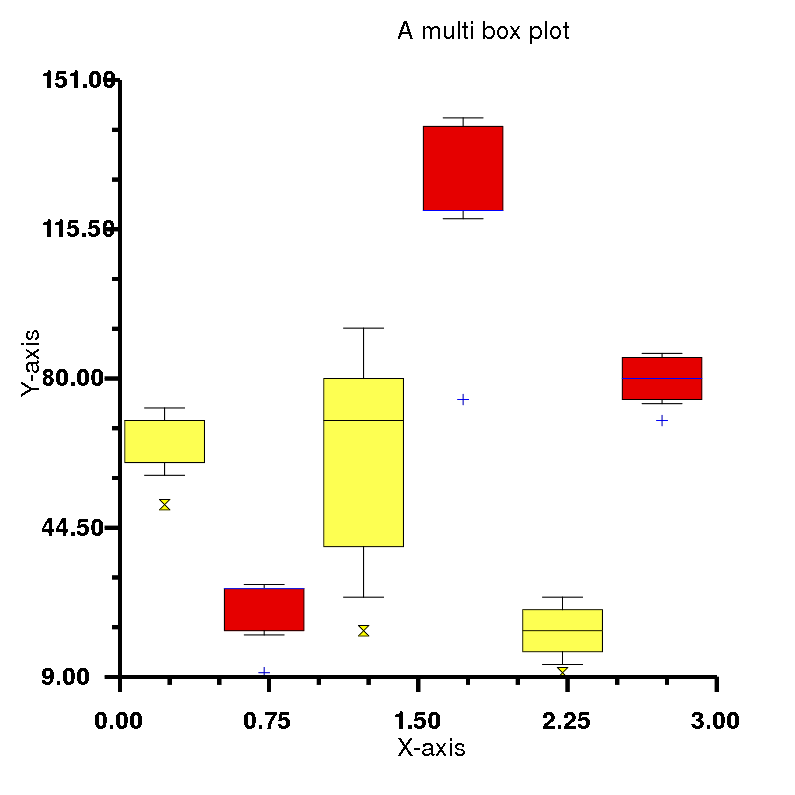### 15. Multiple Subplots Example 1¶

In :
# Overlap of X axis title and the title will be corrected

# 2x2 Subplots
# |------------|------------|
# |            |            |
# |   (1,0)    |    (1,1)   |
# |            |            |
# |------------|------------|
# |            |            |
# |   (0,0)    |    (0,1)   |
# |            |            |
# |------------|------------|

# Step 1
require 'rubyplot'
Rubyplot.set_backend :magick
Rubyplot.inline

# Step 2
figure = Rubyplot::Figure.new(width: 20, height: 20)

# Step 3
axes00.scatter! do |p|
p.data 5.times.map{ rand(100) },5.times.map{ rand(100) } # Data as x_values, y_values
p.marker_type = :triangle_up # Type of marker
p.marker_fill_color = :lemon # Colour to be filled inside the marker
p.marker_size = 4 # Size of the marker, unit is 15*pixels
p.marker_border_color = :black # Colour of the border of the marker
p.label = "Traingle"# Label for this data
end
axes00.title = "A traingle scatter plot"
axes00.x_title = "X-axis"
axes00.y_title = "Y-axis"
axes00.square_axes = false

axes01.scatter! do |p|
p.data 5.times.map{ rand(100) },5.times.map{ rand(100) } # Data as x_values, y_values
p.marker_type = :hglass # Type of marker
p.marker_fill_color = :silver # Colour to be filled inside the marker
p.marker_size = 4 # Size of the marker, unit is 15*pixels
p.marker_border_color = :black # Colour of the border of the marker
p.label = "Hourglass"# Label for this data
end
axes01.title = "A hourglass scatter plot"
axes01.x_title = "X-axis"
axes01.y_title = "Y-axis"
axes01.square_axes = false

axes10.scatter! do |p|
p.data 5.times.map{ rand(100) },5.times.map{ rand(100) } # Data as x_values, y_values
p.marker_type = :diagonal_cross # Type of marker
p.marker_fill_color = :red # Colour to be filled inside the marker
p.marker_size = 4 # Size of the marker, unit is 15*pixels
p.marker_border_color = :black # Colour of the border of the marker
p.label = "Cross"# Label for this data
end
axes10.title = "A cross scatter plot"
axes10.x_title = "X-axis"
axes10.y_title = "Y-axis"
axes10.square_axes = false

axes11.scatter! do |p|
p.data 5.times.map{ rand(100) },5.times.map{ rand(100) } # Data as x_values, y_values
p.marker_type = :solid_plus # Type of marker
p.marker_fill_color = :yellow # Colour to be filled inside the marker
p.marker_size = 4 # Size of the marker, unit is 15*pixels
p.marker_border_color = :black # Colour of the border of the marker
p.label = "Plus"# Label for this data
end
axes11.title = "A plus scatter plot"
axes11.x_title = "X-axis"
axes11.y_title = "Y-axis"
axes11.square_axes = false

# Step 4
figure.show

Out: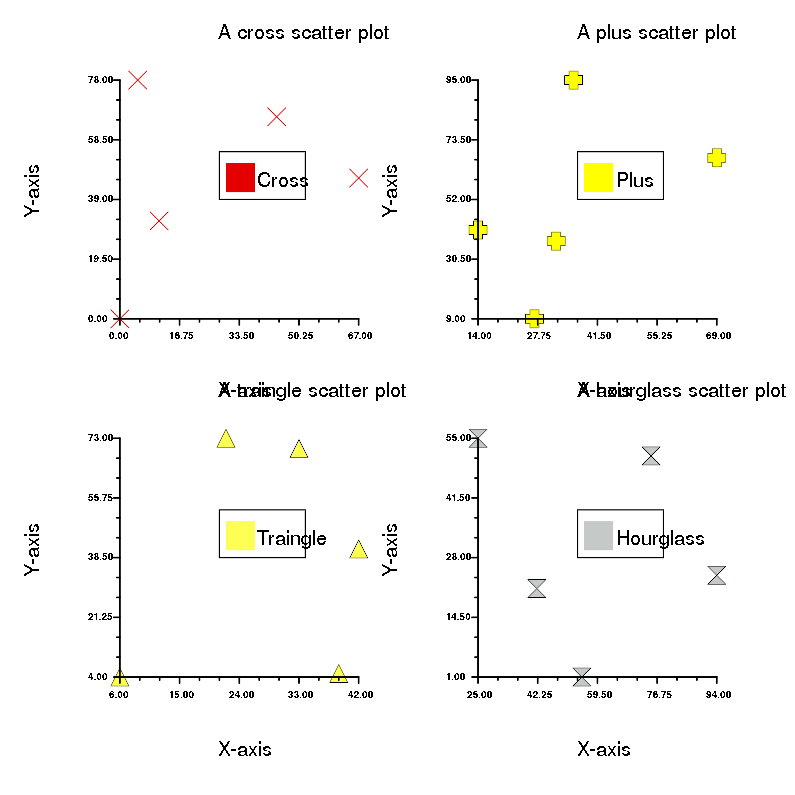### 16. Multiple Subplots Example 2¶

In :
# Overlap of X axis title and the title will be corrected

# 3x2 Subplots
# |------------|------------|
# |            |            |
# |   (2,0)    |    (2,1)   |
# |            |            |
# |------------|------------|
# |            |            |
# |   (1,0)    |    (1,1)   |
# |            |            |
# |------------|------------|
# |            |            |
# |   (0,0)    |    (0,1)   |
# |            |            |
# |------------|------------|

# Step 1
require 'rubyplot'
Rubyplot.set_backend :magick
Rubyplot.inline

# Step 2
figure = Rubyplot::Figure.new(width: 20, height: 30)

# Step 3
axes00.scatter! do |p|
p.data 5.times.map{ rand(100) },5.times.map{ rand(100) } # Data as x_values, y_values
p.marker_type = :diamond # Type of marker
p.marker_fill_color = :lemon # Colour to be filled inside the marker
p.marker_size = 5 # Size of the marker, unit is 15*pixels
p.marker_border_color = :black # Colour of the border of the marker
p.label = "Diamonds" # Label for this data
end
axes00.title = "A scatter plot"
axes00.x_title = "X-axis"
axes00.y_title = "Y-axis"
axes00.square_axes = false

axes01.histogram! do |p|
p.data 100.times.map{ rand(10) } # Data as an array of values
p.color = :electric_lime # Colour of the bars
p.label = "Counts" # Label for this data
# bins are not given so they are decided by Rubyplot
end
axes01.title = "A histogram"
axes01.x_title = "X-axis"
axes01.y_title = "Y-axis"
axes01.square_axes = true

axes10.error_bar! do |p|
p.data [1,2,3,4], [1,4,9,16] # Arrays for x coordinates and y coordinates
p.xerr = [0.5,1.0,1.5,0.3] # X error for each point
p.yerr = [0.6,0.2,0.8,0.1] # Y error for each point
p.color = :red # Colour of the line
p.label = "Values" # Label for this data
end
axes10.title = "A error-bar plot"
axes10.x_title = "X-axis"
axes10.y_title = "Y-axis"
axes10.square_axes = false

axes11.candle_stick! do |p|
p.lows = [100, 110, 120, 130, 120, 110] # Array for minimum values for sticks
p.highs = [140, 150, 160, 170, 160, 150] # Array for maximum value for sticks
p.opens = [110, 120, 130, 140, 130, 120] # Array for minimum value for bars
p.closes = [130, 140, 150, 160, 150, 140] # Array for maximum value for bars
p.border_color = :black # Colour of the border of the bars
p.color = :yellow # Colour of the bars
p.label = "Data 1" # Label for this data
end
axes11.candle_stick! do |p|
p.lows = [105, 105, 125, 130, 110, 110] # Array for minimum values for sticks
p.highs = [150, 140, 165, 170, 180, 160] # Array for maximum value for sticks
p.opens = [110, 120, 130, 140, 130, 120] # Array for minimum value for bars
p.closes = [135, 135, 145, 160, 175, 150] # Array for maximum value for bars
p.border_color = :red # Colour of the border of the bars
p.color = :blue # Colour of the bars
p.label = "Data 2" # Label for this data
end
axes11.title = "A multi candle-stick plot"
axes11.x_title = "X-axis"
axes11.y_title = "Y-axis"
axes11.square_axes = true

axes20.line! do |p|
p.data [1, 2, 3, 4, 5],[12, 55, 4, 10, 24] # Data as arrays for values of x coordinates, y coordinates
p.line_type = :solid # Type of the line
p.line_color = :blue # Colour of the line
p.line_width = 2 # Width of the line
p.label = "Values" # Label for this data
end
axes20.title = "A line plot"
axes20.x_title = "X-axis"
axes20.y_title = "Y-axis"
axes20.square_axes = false

axes21.scatter! do |p|
p.data 5.times.map{ rand(100) },5.times.map{ rand(100) } # Data as x_values, y_values
p.marker_type = :solid_bowtie # Type of marker
p.marker_fill_color = :black # Colour to be filled inside the marker
p.marker_size = 3 # Size of the marker, unit is 15*pixels
p.marker_border_color = :black # Colour of the border of the marker
p.label = "Bowtie" # Label for this data
end
axes21.title = "A scatter plot"
axes21.x_title = "X-axis"
axes21.y_title = "Y-axis"
axes21.square_axes = false

# Step 4
figure.show

Out: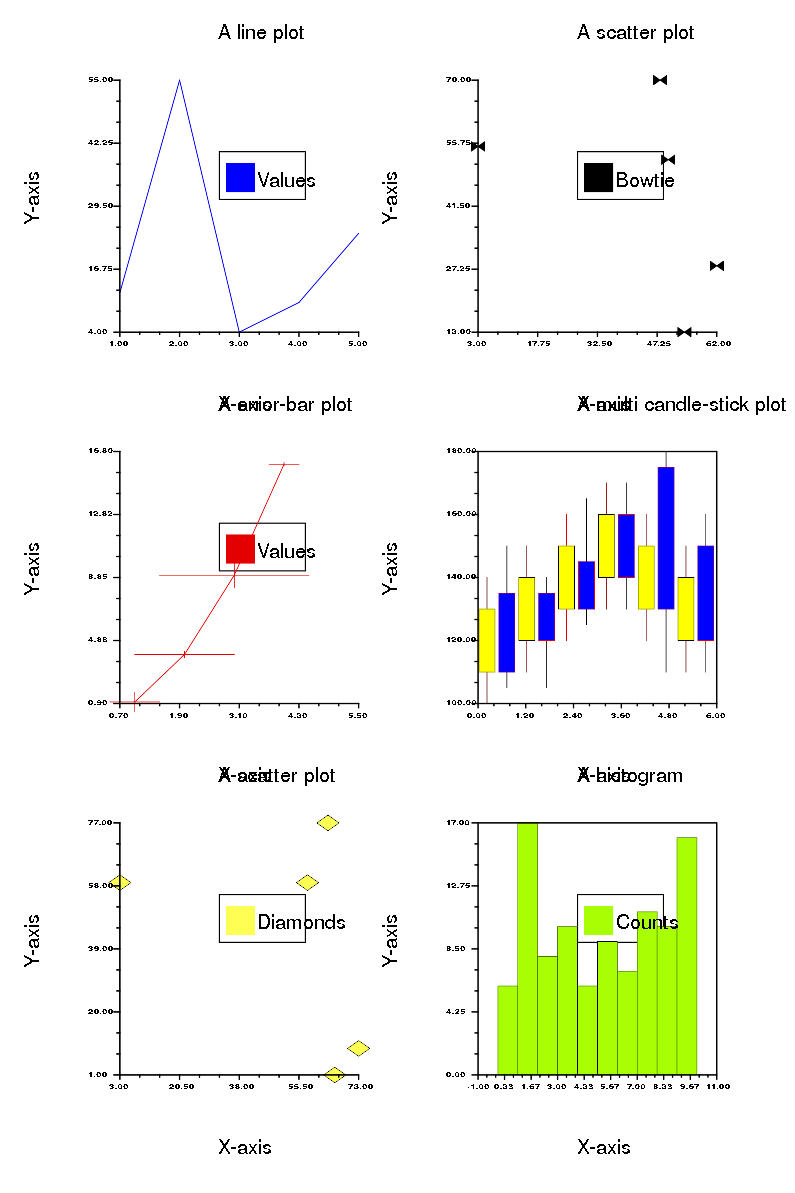### 17. Multiple Subplots Example 3¶

In :
# Overlap of X axis title and the title will be corrected

# 2x3 Subplots
# |------------|------------|------------|
# |            |            |            |
# |   (1,0)    |    (1,1)   |   (1,2)    |
# |            |            |            |
# |------------|------------|------------|
# |            |            |            |
# |   (0,0)    |    (0,1)   |    (0,2)   |
# |            |            |            |
# |------------|------------|------------|

# Step 1
require 'rubyplot'
Rubyplot.set_backend :magick
Rubyplot.inline

# Step 2
figure = Rubyplot::Figure.new(width: 30, height: 20)

# Step 3
axes00.stacked_bar! do |p|
p.data [1, 2, 3, 4, 5] # Data as height of bars
p.color = :lemon # Colour of the bars
p.spacing_ratio = 0.2 # Ratio of space the bars don't occupy out of the maximum space allotted to each bar
# Each bar is allotted equal space, so maximum space for each bar is total space divided by the number of bars
p.label = "Stock 1" # Label for this data
end
# Spacing ratio declared first is considered
axes00.stacked_bar! do |p|
p.data [5, 4, 3, 2, 1] # Data as height of bars
p.color = :blue # Colour of the bars
p.spacing_ratio = 0.2 # Ratio of space the bars don't occupy out of the maximum space allotted to each bar
# Each bar is allotted equal space, so maximum space for each bar is total space divided by the number of bars
p.label = "Stock 2" # Label for this data
end
axes00.stacked_bar! do |p|
p.data [3, 5, 7, 5, 3] # Data as height of bars
p.color = :red # Colour of the bars
p.spacing_ratio = 0.2 # Ratio of space the bars don't occupy out of the maximum space allotted to each bar
# Each bar is allotted equal space, so maximum space for each bar is total space divided by the number of bars
p.label = "Stock 3" # Label for this data
end
axes00.title = "A multi stacked-bar plot"
axes00.x_title = "X-axis"
axes00.y_title = "Y-axis"
axes00.square_axes = false

axes01.line! do |p|
p.data [1, 2, 3, 4, 5],[15, 25, 3, 10, 1] # Data as arrays for values of x coordinates, y coordinates
p.line_type = :solid # Type of the line
p.line_color = :green # Colour of the line
p.line_width = 20 # Width of the line
p.label = "Values" # Label for this data
end
axes01.title = "A line plot"
axes01.x_title = "X-axis"
axes01.y_title = "Y-axis"
axes01.square_axes = false

axes02.area! do |p|
p.data [1, 2, 3, 4, 5, 6], [10, 8, 4, 2, 3, 1] # Data as x coordinate values, height of consecutive points i.e. y coordinates
p.color = :orange # Color of the area
p.label = "Stock A" # Label for this data
p.stacked true # stacked option makes area plot opaque i.e. opacity = 1
# Opacity of the area plot is set to 0.3 for visibility if not stacked
end
axes02.title = "A area plot"
axes02.x_title = "X-axis"
axes02.y_title = "Y-axis"
axes02.square_axes = false

axes10.bar! do |p|
p.data [1, 2, 3, 4, 5] # Data as height of bars
p.color = :lemon # Colour of the bars
p.spacing_ratio = 0.2 # Ratio of space the bars don't occupy out of the maximum space allotted to each bar
# Each bar is allotted equal space, so maximum space for each bar is total space divided by the number of bars
p.label = "Stock 1" # Label for this data
end
# Spacing ratio declared first is considered
axes10.bar! do |p|
p.data [5, 4, 3, 2, 1] # Data as height of bars
p.color = :blue # Colour of the bars
p.spacing_ratio = 0.2 # Ratio of space the bars don't occupy out of the maximum space allotted to each bar
# Each bar is allotted equal space, so maximum space for each bar is total space divided by the number of bars
p.label = "Stock 2" # Label for this data
end
axes10.bar! do |p|
p.data [3, 5, 7, 5, 3] # Data as height of bars
p.color = :red # Colour of the bars
p.spacing_ratio = 0.2 # Ratio of space the bars don't occupy out of the maximum space allotted to each bar
# Each bar is allotted equal space, so maximum space for each bar is total space divided by the number of bars
p.label = "Stock 3" # Label for this data
end
axes10.title = "A multi bar plot"
axes10.x_title = "X-axis"
axes10.y_title = "Y-axis"
axes10.square_axes = false

axes11.bubble! do |p|
p.data [12, 4, 25, 7, 19], [50, 30, 75, 12, 25], [0.5, 0.7, 0.4, 0.5, 1] # Data as arrays of x coordinates, y coordinates and sizes
# Size units are 27.5*pixel
p.color = :blue # Colour of the bubbles
p.label = "Bubbles 1" # Label for this data
# Opacity of the bubbles is set to 0.5 for visibility
end
axes11.bubble! do |p|
p.data [1, 7, 20, 27, 17], [41, 30, 48, 22, 5], [0.5, 1, 0.8, 0.9, 1] # Data as arrays of x coordinates, y coordinates and sizes
# Size units are 27.5*pixel
p.color = :red # Colour of the bubbles
p.label = "Bubbles 2" # Label for this data
# Opacity of the bubbles is set to 0.5 for visibility
end
axes11.title = "A bubble plot"
axes11.x_title = "X-axis"
axes11.y_title = "Y-axis"
axes11.square_axes = false

axes12.box_plot! do |p|
p.data [
[60,70,80,70,50],
[100,40,20,80,70],
[30, 10]
] # Array of arrays for data for each box
p.color = :blue # Colours of the boxes
p.whiskers = 0.3 # whiskers for determining outliers
p.outlier_marker_type = :hglass # Type of the outlier marker
p.outlier_marker_color = :yellow # Fill colour of the outlier marker
# Border colour of the outlier marker is set to black
p.outlier_marker_size = 1 # Size of the outlier marker
p.label = "Data" # Label for this data
end
axes12.title = "A box plot"
axes12.x_title = "X-axis"
axes12.y_title = "Y-axis"
axes12.square_axes = false

# Step 4
figure.show

Out: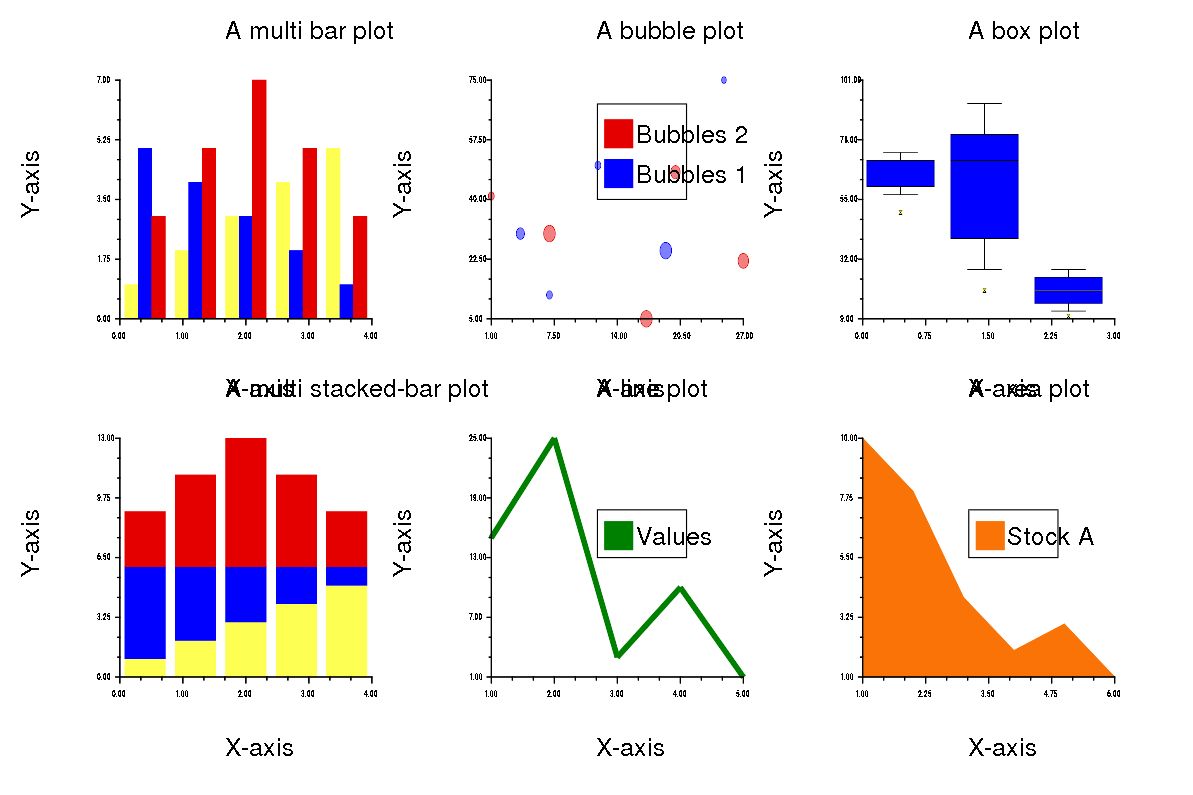### 18. Plot function Examples¶

#### Only line¶

In :
# Default for plot function is solid black line

# Step 1
require 'rubyplot'
Rubyplot.set_backend :magick
Rubyplot.inline

# Step 2
figure = Rubyplot::Figure.new(width: 20, height: 20)

# Step 3
axes00.plot! do |p|
d = (0..360).step(5).to_a
p.data d, d.map { |a| Math.sin(a * Math::PI / 180) } # Data as arrays of x coordinates and y coordinates
#   p.marker_type = :diamond # Type of marker
#   p.marker_fill_color = :lemon # Colour to be filled inside the marker
#   p.marker_size = 2 # Size of the marker, unit is 15*pixels
#   p.marker_border_color = :black # Colour of the border of the marker
p.line_type = :solid # Type of the line
p.line_color = :black # Colour of the line
p.line_width = 2 # Width of the line
#   p.fmt = 'b.-' # fmt argument to specify line type, marker type and colour in short
# fmt argument overwrites line type, marker type and all the colours i.e. marker_fill_color, marker_border_color, line_color
# line type, marker type and colour can be in any order
p.label = "sine" # Label for this data
end

axes00.title = "A plot function example"
axes00.x_title = "X-axis"
axes00.y_title = "Y-axis"
axes00.square_axes = false

# Step 4
figure.show

Out: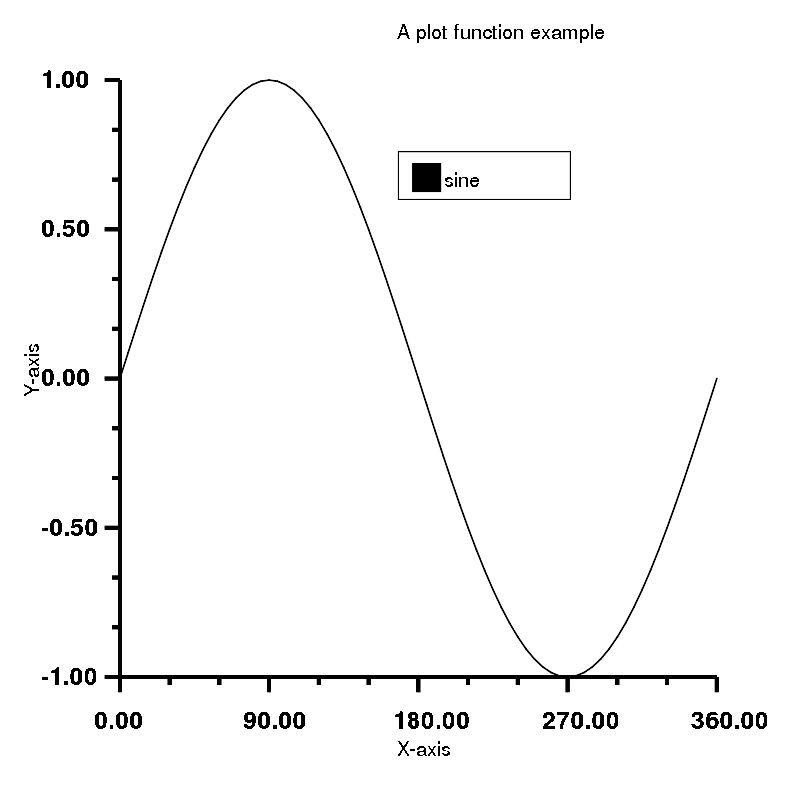#### Only markers¶

In :
# Colour of the legend will be corrected
# Default for plot function is solid black line

# Step 1
require 'rubyplot'
Rubyplot.set_backend :magick
Rubyplot.inline

# Step 2
figure = Rubyplot::Figure.new(width: 20, height: 20)

# Step 3
axes00.plot! do |p|
d = (0..360).step(5).to_a
p.data d, d.map { |a| Math.sin(a * Math::PI / 180) } # Data as arrays of x coordinates and y coordinates
p.marker_type = :plus # Type of marker
p.marker_fill_color = :blue # Colour to be filled inside the marker
p.marker_size = 1 # Size of the marker, unit is 15*pixels
p.marker_border_color = :blue # Colour of the border of the marker
#   p.line_type = :solid # Type of the line
#   p.line_color = :black # Colour of the line
#   p.line_width = 2 # Width of the line
#   p.fmt = 'b.-' # fmt argument to specify line type, marker type and colour in short
# fmt argument overwrites line type, marker type and all the colours i.e. marker_fill_color, marker_border_color, line_color
# line type, marker type and colour can be in any order
p.label = "sine" # Label for this data
end

axes00.title = "A plot function example"
axes00.x_title = "X-axis"
axes00.y_title = "Y-axis"
axes00.square_axes = false

# Step 4
figure.show

Out: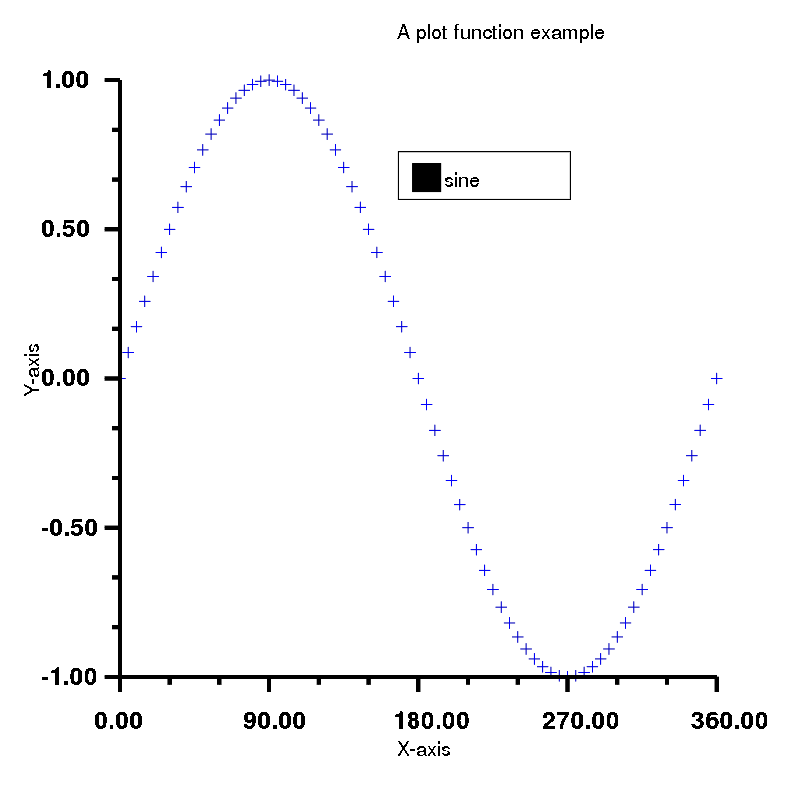#### Both line and markers¶

In :
# Default for plot function is solid black line

# Step 1
require 'rubyplot'
Rubyplot.set_backend :magick
Rubyplot.inline

# Step 2
figure = Rubyplot::Figure.new(width: 20, height: 20)

# Step 3
axes00.plot! do |p|
d = (0..360).step(5).to_a
p.data d, d.map { |a| Math.sin(a * Math::PI / 180) } # Data as arrays of x coordinates and y coordinates
p.marker_type = :circle # Type of marker
p.marker_fill_color = :white # Colour to be filled inside the marker
p.marker_size = 0.5 # Size of the marker, unit is 15*pixels
p.marker_border_color = :black # Colour of the border of the marker
p.line_type = :solid # Type of the line
p.line_color = :black # Colour of the line
p.line_width = 2 # Width of the line
#   p.fmt = 'b.-' # fmt argument to specify line type, marker type and colour in short
# fmt argument overwrites line type, marker type and all the colours i.e. marker_fill_color, marker_border_color, line_color
# line type, marker type and colour can be in any order
p.label = "sine" # Label for this data
end

axes00.title = "A plot function example"
axes00.x_title = "X-axis"
axes00.y_title = "Y-axis"
axes00.square_axes = false

# Step 4
figure.show

Out: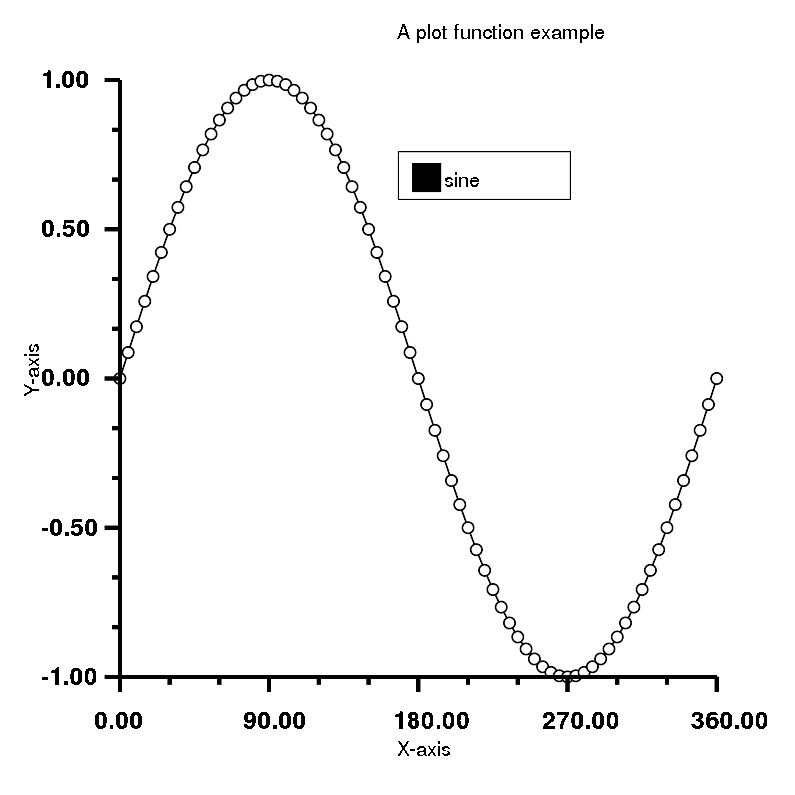#### fmt argument only line¶

In :
# Default for plot function is solid black line

# Step 1
require 'rubyplot'
Rubyplot.set_backend :magick
Rubyplot.inline

# Step 2
figure = Rubyplot::Figure.new(width: 20, height: 20)

# Step 3
axes00.plot! do |p|
d = (0..360).step(1).to_a
p.data d, d.map { |a| Math.sin(a * Math::PI / 180) } # Data as arrays of x coordinates and y coordinates
#   p.marker_type = :diamond # Type of marker
#   p.marker_fill_color = :lemon # Colour to be filled inside the marker
#   p.marker_size = 2 # Size of the marker, unit is 15*pixels
#   p.marker_border_color = :black # Colour of the border of the marker
#   p.line_type = :solid # Type of the line
#   p.line_color = :black # Colour of the line
#   p.line_width = 2 # Width of the line
p.fmt = '-' # fmt argument to specify line type, marker type and colour in short
# fmt argument overwrites line type, marker type and all the colours i.e. marker_fill_color, marker_border_color, line_color
# line type, marker type and colour can be in any order
p.label = "sine" # Label for this data
end

axes00.title = "A plot function example"
axes00.x_title = "X-axis"
axes00.y_title = "Y-axis"
axes00.square_axes = false

# Step 4
figure.show

Out: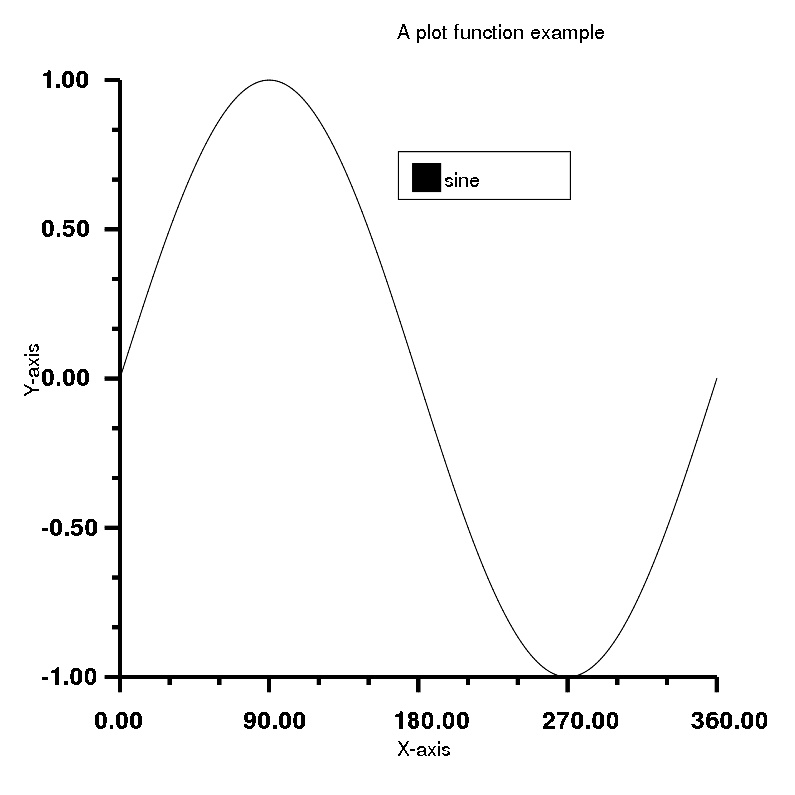#### fmt argument only colour¶

In :
# Default for plot function is solid black line

# Step 1
require 'rubyplot'
Rubyplot.set_backend :magick
Rubyplot.inline

# Step 2
figure = Rubyplot::Figure.new(width: 20, height: 20)

# Step 3
axes00.plot! do |p|
d = (0..360).step(1).to_a
p.data d, d.map { |a| Math.sin(a * Math::PI / 180) } # Data as arrays of x coordinates and y coordinates
#   p.marker_type = :diamond # Type of marker
#   p.marker_fill_color = :lemon # Colour to be filled inside the marker
#   p.marker_size = 2 # Size of the marker, unit is 15*pixels
#   p.marker_border_color = :black # Colour of the border of the marker
#   p.line_type = :solid # Type of the line
#   p.line_color = :black # Colour of the line
#   p.line_width = 2 # Width of the line
p.fmt = 'r' # fmt argument to specify line type, marker type and colour in short
# fmt argument overwrites line type, marker type and all the colours i.e. marker_fill_color, marker_border_color, line_color
# line type, marker type and colour can be in any order
p.label = "sine" # Label for this data
end

axes00.title = "A plot function example"
axes00.x_title = "X-axis"
axes00.y_title = "Y-axis"
axes00.square_axes = false

# Step 4
figure.show

Out: